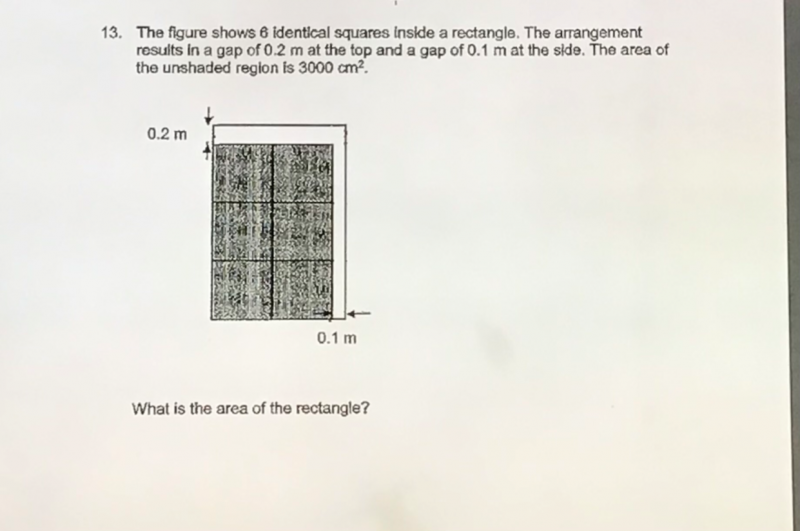# QuestionAlternative Solution:

Let u be the side of the square.

0.2m = 20 cm,  0.1m = 10cm

20(2u+10) + 10(3u) = 3000

40u+200+30u = 3000

70u=2800

u=40

Therefore area of rectangle = 2u x 3u = 2(40) x 3(40) = 80 x 120 = 9600 cm2

0 Replies 1 Like